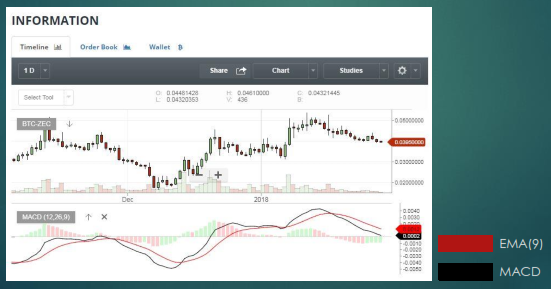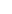# Guide for traders

MACD (Moving Average Convergence - Divergence) is formed by the difference between two different periods of exponential moving averages (EMA). In particular, it is calculated by deducting from a long-term EMA (26 periods) a one short-term EMA (12 periods). The MACD depiction is made along with another EMA of 9 periods this time, also called the Signal Line. In summary, we can say that when MACD breaks up the Signal Line, then we can buy, and when MACD breaks down the Signal Line, we can sell. Depending on how volatile the price of a coin is, we set the EMA periods. The more volatile the price of a coin, the better it is to use fewer periods.---to signal pageMoving Average Convergence – Divergence (MACD) ❺❺❺ - average rating 5 from 5 (based on 40 user reviews)